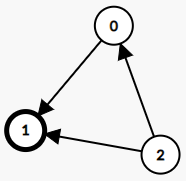277. Find the Celebrity

Suppose you are at a party with `n` people (labeled from `0` to `n - 1`), and among them, there may exist one celebrity. The definition of a celebrity is that all the other `n - 1` people know him/her, but he/she does not know any of them.

Now you want to find out who the celebrity is or verify that there is not one. The only thing you are allowed to do is to ask questions like: "Hi, A. Do you know B?" to get information about whether A knows B. You need to find out the celebrity (or verify there is not one) by asking as few questions as possible (in the asymptotic sense).

You are given a helper function `bool knows(a, b)` which tells you whether A knows B. Implement a function `int findCelebrity(n)`. There will be exactly one celebrity if he/she is in the party. Return the celebrity's label if there is a celebrity in the party. If there is no celebrity, return `-1`.

Example 1:```Input: graph = [[1,1,0],[0,1,0],[1,1,1]]
Output: 1
Explanation: There are three persons labeled with 0, 1 and 2. graph[i][j] = 1 means person i knows person j, otherwise graph[i][j] = 0 means person i does not know person j. The celebrity is the person labeled as 1 because both 0 and 2 know him but 1 does not know anybody.
```

Example 2:```Input: graph = [[1,0,1],[1,1,0],[0,1,1]]
Output: -1
Explanation: There is no celebrity.
```

Constraints:

• `n == graph.length`
• `n == graph[i].length`
• `2 <= n <= 100`
• `graph[i][j]` is `0` or `1`.
• `graph[i][i] == 1`

277. Find the Celebrity
``````struct Solution {
n: usize,
}

impl Solution {
fn new(graph: Vec<Vec<i32>>) -> Self {
let n = graph.len();
let mut adj = vec![vec![false; n]; n];
for i in 0..n {
for j in 0..n {
}
}
}

fn knows(&self, a: i32, b: i32) -> bool {
}
}

impl Solution {
fn find_celebrity(&self, n: i32) -> i32 {
let n = n as usize;
for i in 0..n {
let mut x = 0;
let mut y = 0;
for j in 0..n {
if i != j {
if self.knows(i as i32, j as i32) {
x += 1;
}
if self.knows(j as i32, i as i32) {
y += 1;
}
}
}
if x == 0 && y == n - 1 {
return i as i32;
}
}
-1
}
}

#[test]
fn test() {
let graph = vec_vec_i32![[1, 1, 0], [0, 1, 0], [1, 1, 1]];
let solution = Solution::new(graph);
let n = 3;
let res = 1;
assert_eq!(solution.find_celebrity(n), res);
let graph = vec_vec_i32![[1, 0, 1], [1, 1, 0], [0, 1, 1]];
let solution = Solution::new(graph);
let n = 3;
let res = -1;
assert_eq!(solution.find_celebrity(n), res);
}
``````